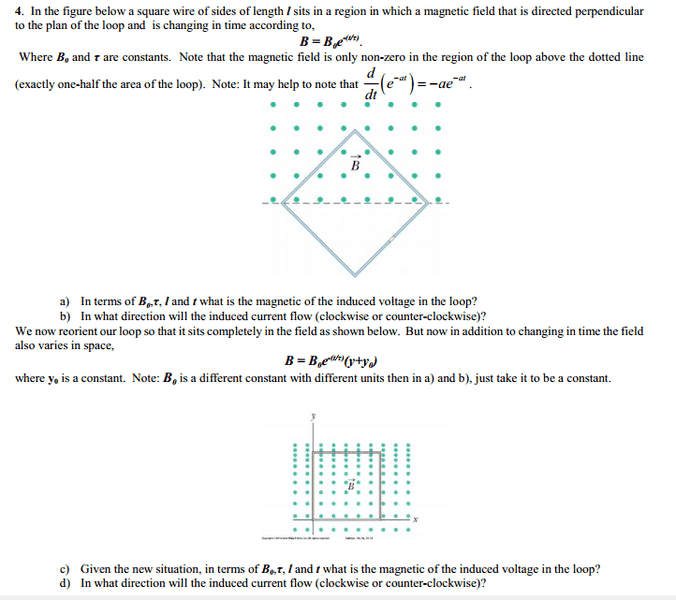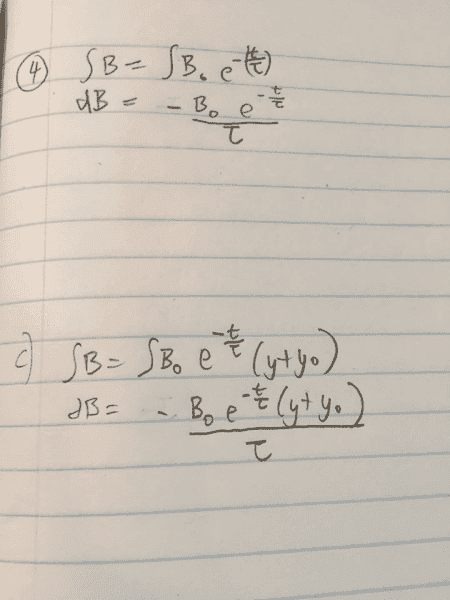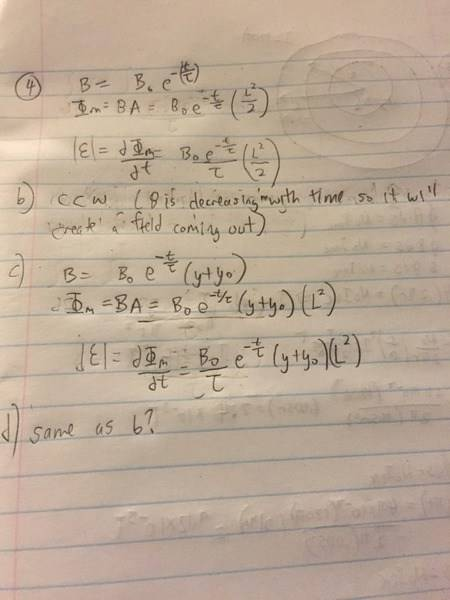# Magnitude and Direction of induced voltage

• rzn972
In summary, the conversation is about a physics e&m question involving finding the magnitude of the induced voltage using Faraday's Law and Lenz's Law. There is a discussion about whether to include a constant "c" and determining the direction of the current flow using the right hand rule. The conversation also includes a calculation of flux and determining the area of the shape in question. There is a brief discussion about accounting for different flux values and the direction of the current.

## Homework Statement

Hey everyone I have a physics e&m question: [/B]
http://blob:https%3A//www.physicsforums.com/aa779f37-be23-43d7-a888-d70263a3854b## The Attempt at a SolutionI took the derivative to find the magnitude of the induced voltage. (do I need to add a constant c?)
I am having trouble with the direction of the current flow though... Do I use the right hand rule?

Thanks![/B]

#### Attachments

rzn972 said:
I took the derivative to find the magnitude of the induced voltage. (do I need to add a constant c?)
I am having trouble with the direction of the current flow though... Do I use the right hand rule?
The constant "c" is usually either the speed of light in a vacuum or the constant of integration ... do either of these situation apply here?
The current flow is determined by the right hand rule in conjunction with a law of physics that applies to induced currents.
I'm puzzled you started at (c) ... did you do (a) and (b)?

4 a) Does the question ask for the magnetic (flux) ?

Oh, it should read the magnitude, not magnetic!

There might be a small gap in the lower right-hand part of the loop

•Simon Bridge
Well spotted - there may well be.

Sorry! Yeah I meant magnitude.

Last edited:
Okay so the derivative of magnetic flux is the induced voltage. I am having trouble calculating flux. Flux = BA cos (theta). How do I get the area...?

What shape is the area in question?

Ahh I forgot that they gave us the length of the square. I think I was able to get it.Does this look right?

in (c) and (d) the flux density is different in different parts of the square, how did you account for this?